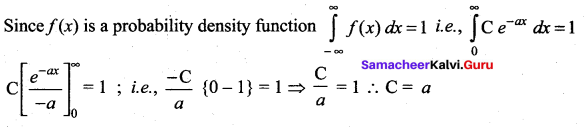# Samacheer Kalvi 12th Maths Solutions Chapter 11 Probability Distributions Ex 11.3

## Tamilnadu Samacheer Kalvi 12th Maths Solutions Chapter 11 Probability Distributions Ex 11.3

Question 1.
The probability density function of X is given by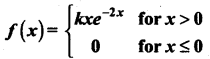Find the value of k.
Solution: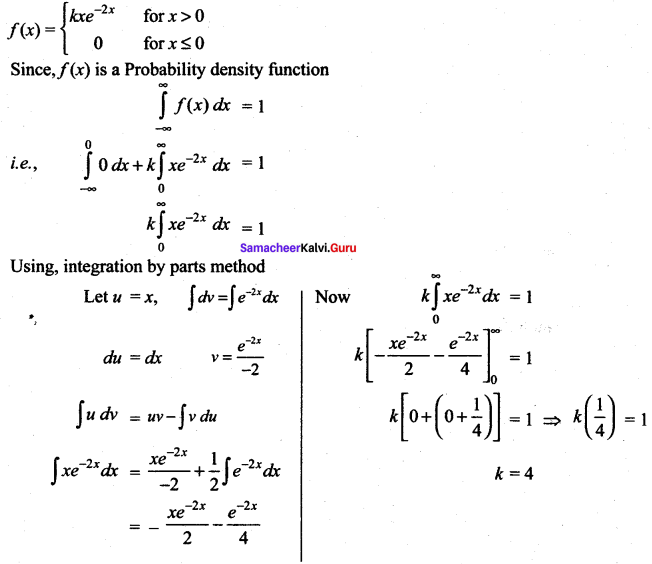Question 2.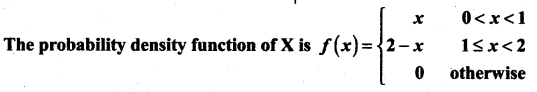Solution: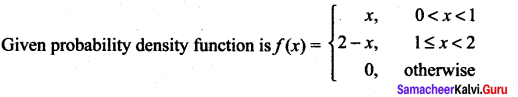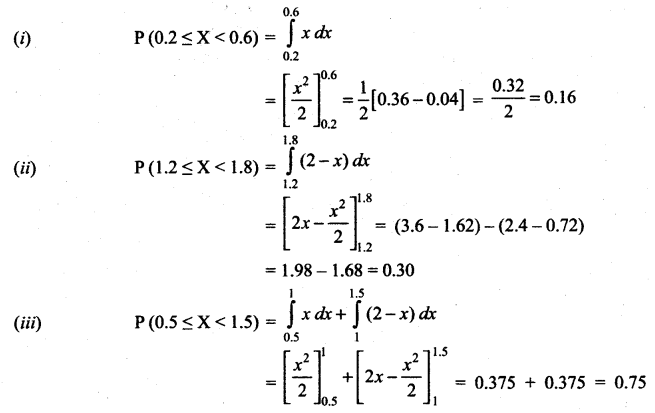Question 3.
Suppose the amount of milk sold daily at a milk booth is distributed with a minimum of 200 litres and a maximum of 600 litres with probability density function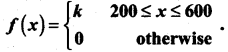Find (i) the value of k
(ii) the distribution function
(iii) the probability that daily sales will fall between 300 litres and 500 litres?
Solution: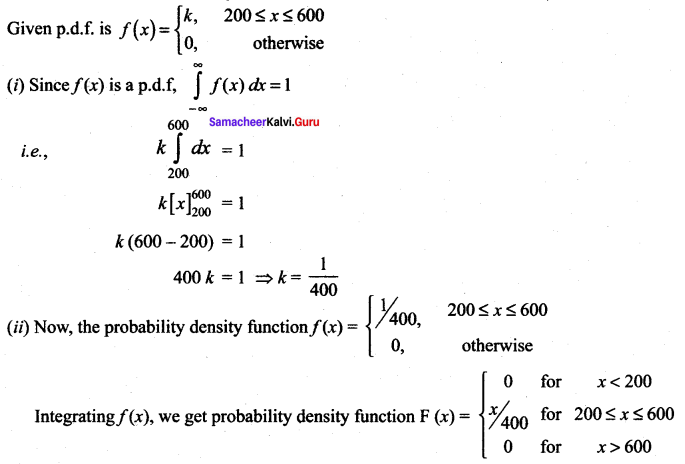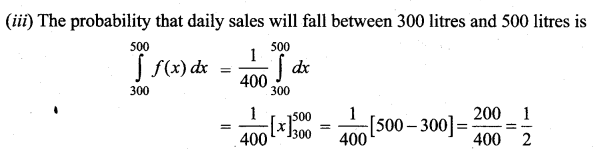Question 4.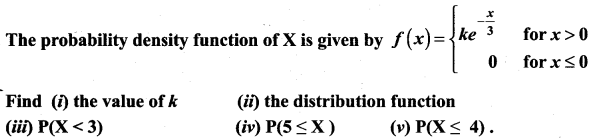Solution: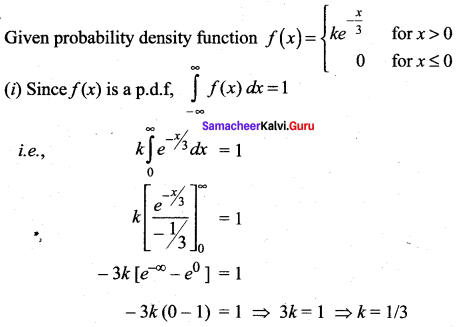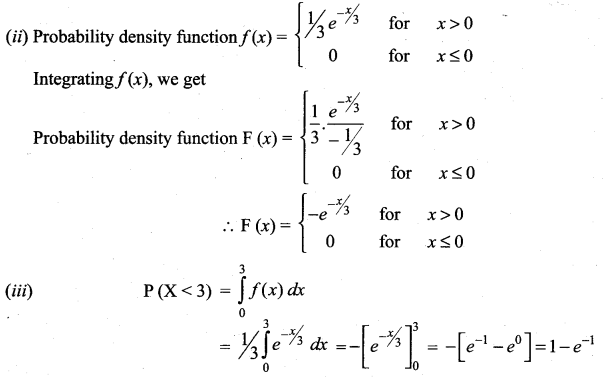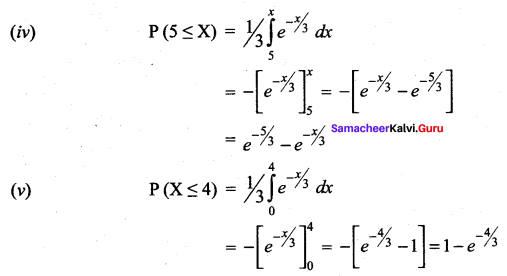Question 5.
If X is a random variable with probability density function f(x) given by,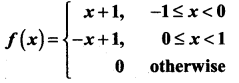Solution: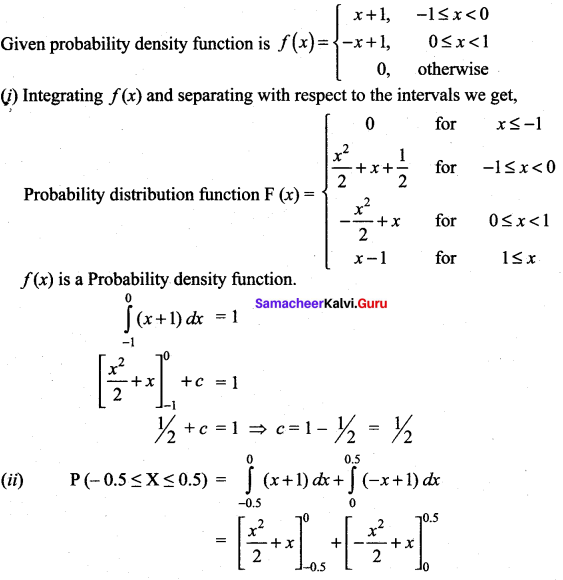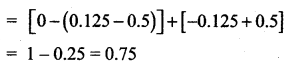Question 6.
If X is the random variable with probability density function F(x) given by,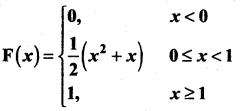then find (i) the distribution function f(x)
(ii) P(0.3 ≤ X ≤ 0.6)
Solution: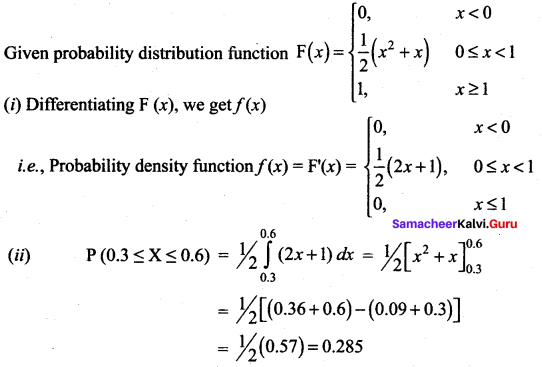### Samacheer Kalvi 12th Maths Solutions Chapter 11 Probability Distributions Ex 11.3 Additional Problems

Question 1.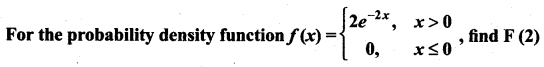Solution: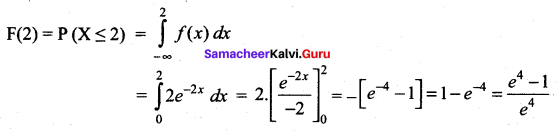Question 2.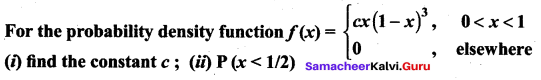Solution: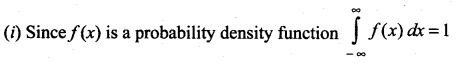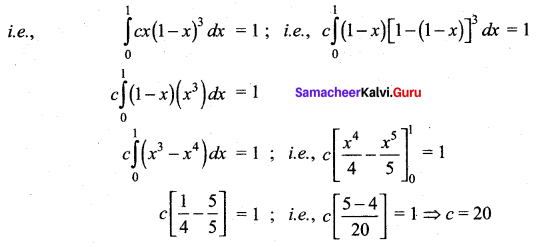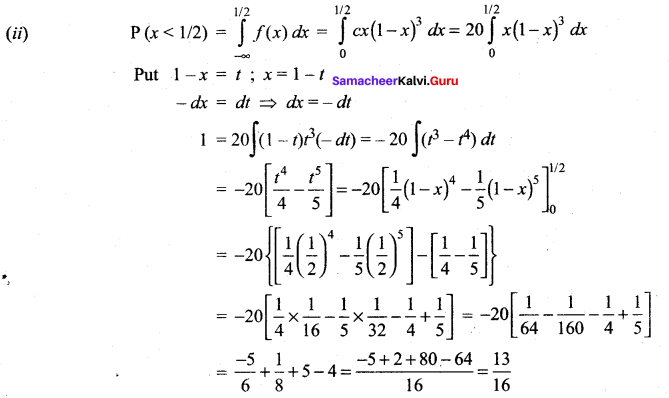Question 3.
The probability density function of a random variable x is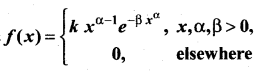Find
(i) k;
(ii) P(X > 10)
Solution: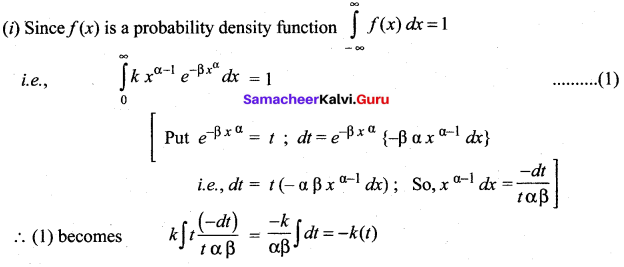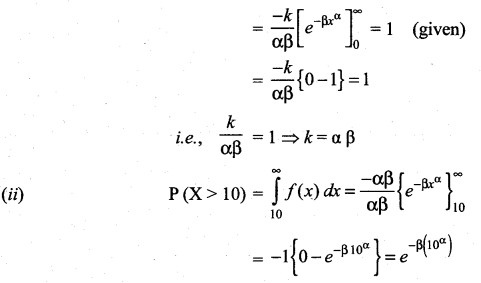Question 4.
A continuous random variable x has the p.d.f. defined by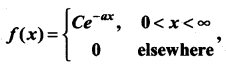find the value of C if a > 0.
Solution: